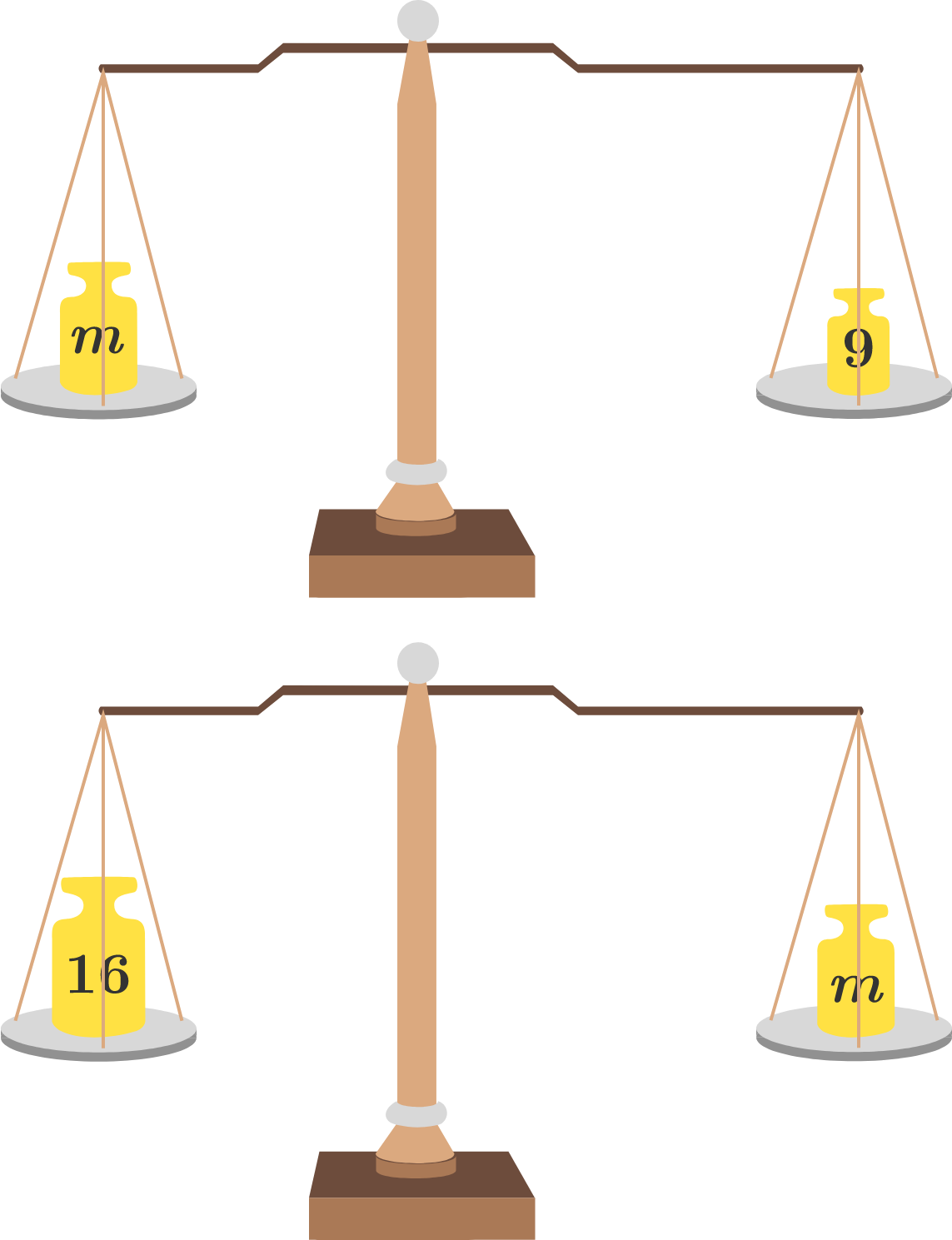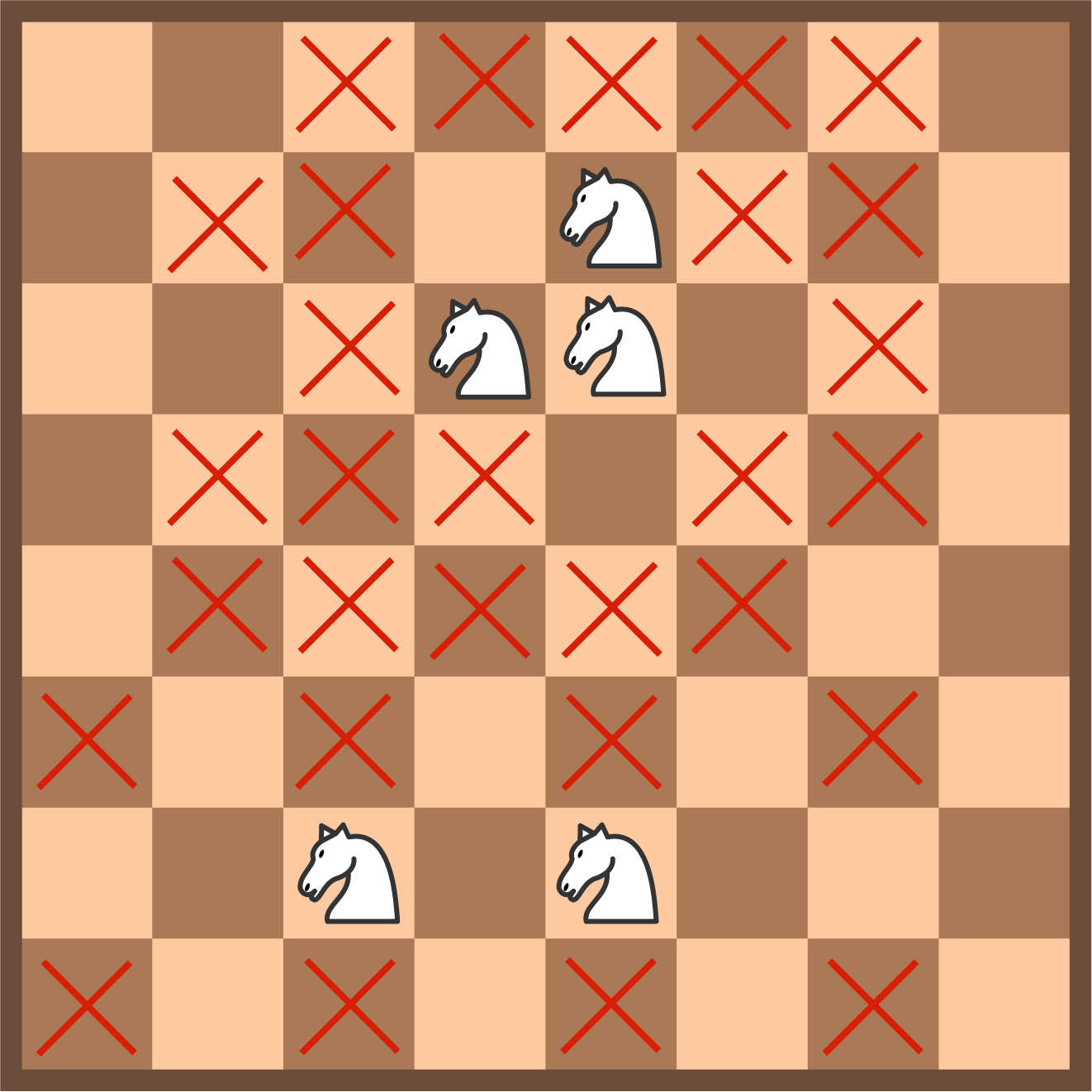# Problems of the Week

Contribute a problem

# 2017-02-06 Intermediate

A positive integer $n$ leaves the same remainder of 35 when divided by both 2009 and 2010.

What is the remainder when $n$ is divided by 42?A faulty beam balance has pans of different masses, and its beam remains horizontal when the pans are empty. On such a beam balance, a mass of $m$ is put on one pan and it is balanced by a weight of 9 grams. When the same mass is put on the other pan, it can be balanced by a weight of 16 grams.

Find the mass $m$ in grams.

The following is my attempt at proving that $1 < -1$. In which of these steps did I first make a mistake by using flawed logic?

Step 1: Let $i = \sqrt{-1}$, then $i^2 = -1$ and $i^4 = \big(i^2\big)^2 = (-1)^2 = 1$.

Step 2: Hence, we have $i^2 < i^4$.

Step 3: We divide both sides by $i$ to get $i < i^3$.

Step 4: We divide both sides by $i$ again to get $1 < i^2$ or, equivalently, $1 < -1$.Andrew and Benjamin are playing a game on an $8 \times 8$ chessboard. Each turn, they place a knight in a position that isn't threatened by other knights that are already on the board. The first person who is unable to place a knight loses the game.

As an explicit example, the board above shows a possible sequence of 5 turns, where all the squares that are threatened by other knights are marked with red X's.

If Andrew goes first, who will win this game?

$\large \sum_{n=1}^{\infty} \frac{2^{2^{n}}-1}{2^{2^{n+1}}} = \frac ab$

If the equation above holds true for coprime positive integers $a$ and $b$, find the value of $a+b$.

×